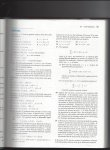Ricatti ODE v. generalized Ricatti ODE; use of Bernouli ODE step (in video)

Metronome

New member
I noticed that in the Ricatti ODE introduction in this video (https://www.youtube.com/watch?v=-SHQ1FMyqIo), the form of the general solution is stated as y = y_1 + u and the Ricatti ODE becomes a Bernoulli ODE, which eventually becomes linear, while in this video (https://www.youtube.com/watch?v=3heIoKDhN3E), the stated general solution is y = y_1 + 1/v, and the Ricatti ODE becomes a linear ODE directly. Is this difference in the assumed general solution form the reason for the presence or absence of the intermediate Bernoulli ODE step? If not, why is the more complex y = y_1 + 1/v sometimes preferred, and what conditions determine whether the Ricatti ODE will become Bernoulli as an intermediate step, or will be linear immediately?

Also, what is the difference in a nutshell between the Ricatti ODE and the generalized Ricatti ODE?

Subhotosh Khan

Super Moderator
Staff member
I noticed that in the Ricatti ODE introduction in this video (https://www.youtube.com/watch?v=-SHQ1FMyqIo), the form of the general solution is stated as y = y_1 + u and the Ricatti ODE becomes a Bernoulli ODE, which eventually becomes linear, while in this video (https://www.youtube.com/watch?v=3heIoKDhN3E), the stated general solution is y = y_1 + 1/v, and the Ricatti ODE becomes a linear ODE directly. Is this difference in the assumed general solution form the reason for the presence or absence of the intermediate Bernoulli ODE step? If not, why is the more complex y = y_1 + 1/v sometimes preferred, and what conditions determine whether the Ricatti ODE will become Bernoulli as an intermediate step, or will be linear immediately?

Also, what is the difference in a nutshell between the Ricatti ODE and the generalized Ricatti ODE?
What research have you done regarding that? What did you find?

Metronome

New member
What research have you done regarding that? What did you find?
This video (https://youtu.be/3heIoKDhN3E?t=43) introducing the Riccati ODE references a more general form than the one it covers. I presume this is a reference to the notably more daunting linear algebraic equations in the Wikipedia article on Linear-Quadradic-Gaussian Control (https://en.wikipedia.org/wiki/Linear–quadratic–Gaussian_control). However, my differential equations textbook attempts to introduce the generalized Riccati ODE without introducing the Riccati ODE.It appears more or less identical to the Riccati ODE found in other sources, so if there are actually three levels of generality, I'm not certain what the less general form than the book's would look like.

Last edited: